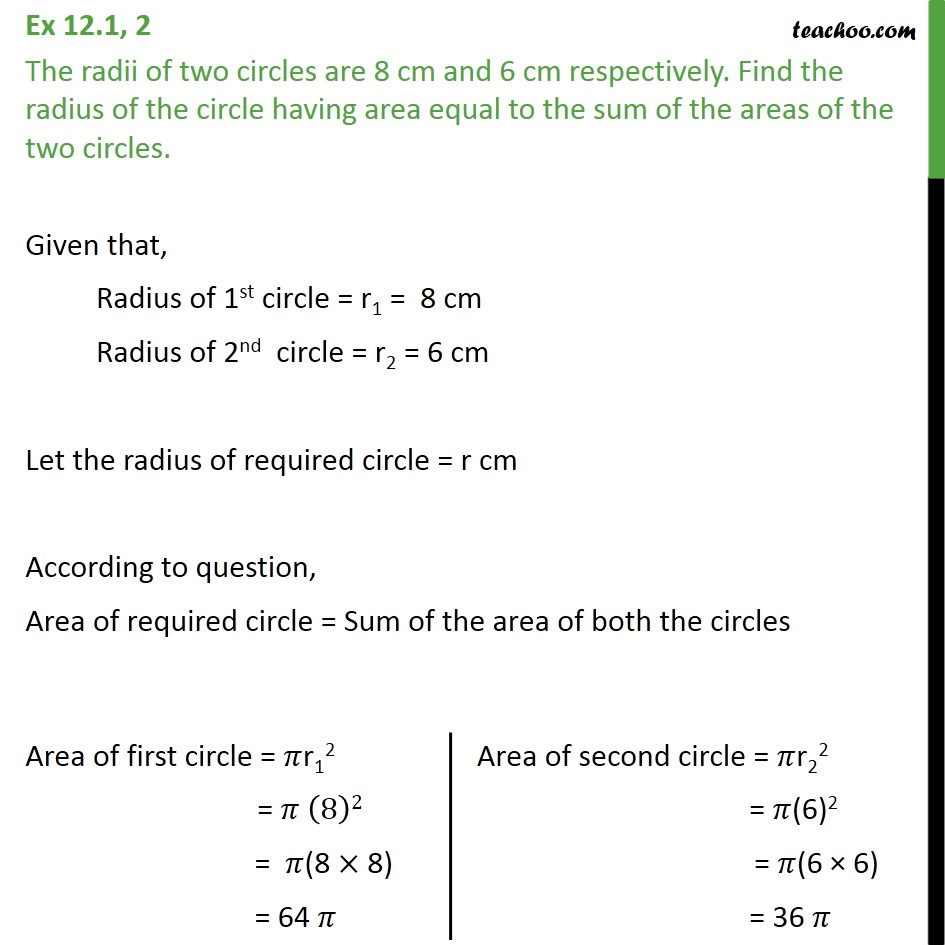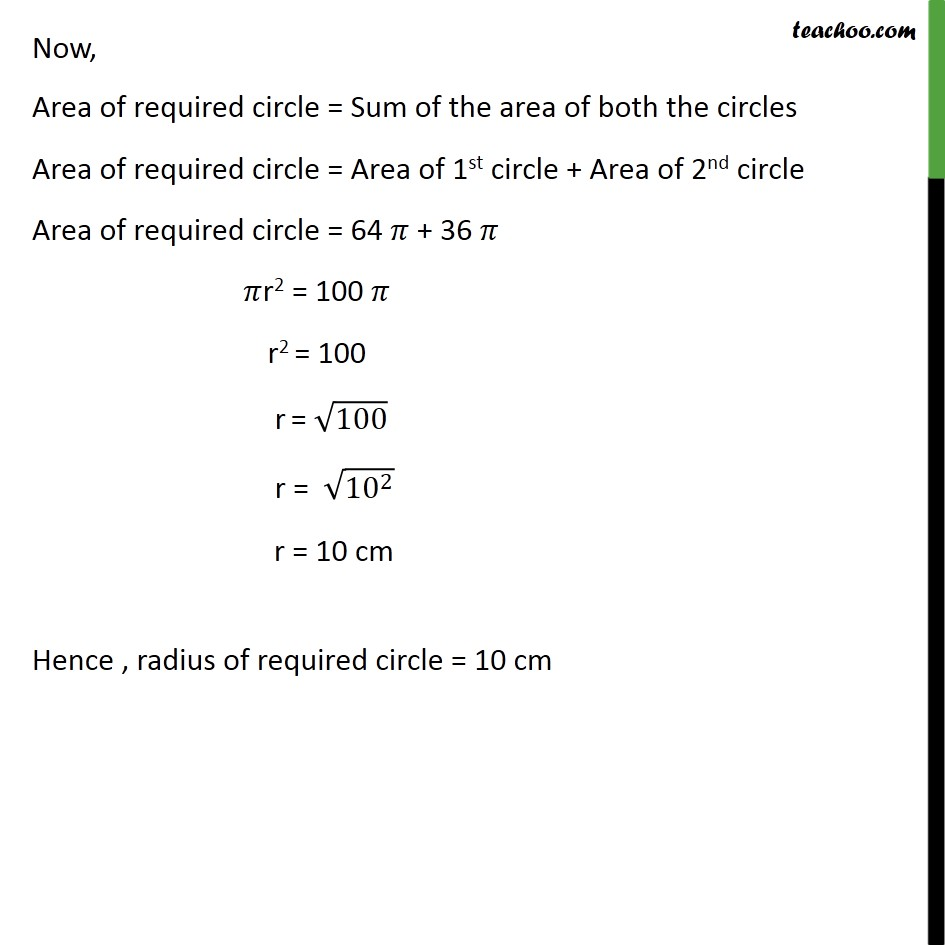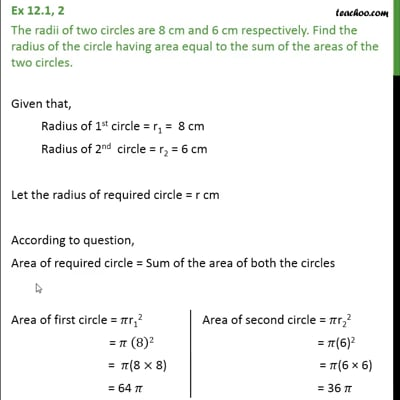Area/Perimeter of Circle

Chapter 12 Class 10 Areas related to Circles
Concept wiseThis video is only available for Teachoo black users

Introducing your new favourite teacher - Teachoo Black, at only ₹83 per month

### Transcript

Ex 12.1, 2 The radii of two circles are 8 cm and 6 cm respectively. Find the radius of the circle having area equal to the sum of the areas of the two circles. Given that, Radius of 1st circle = r1 = 8 cm Radius of 2nd circle = r2 = 6 cm Let the radius of required circle = r cm According to question, Area of required circle = Sum of the area of both the circles Now, Area of required circle = Sum of the area of both the circles Area of required circle = Area of 1st circle + Area of 2nd circle Area of required circle = 64 𝜋 + 36 𝜋 𝜋r2 = 100 𝜋 r2 = 100 r = √100 r = √(〖10〗^2 ) r = 10 cm Hence , radius of required circle = 10 cm